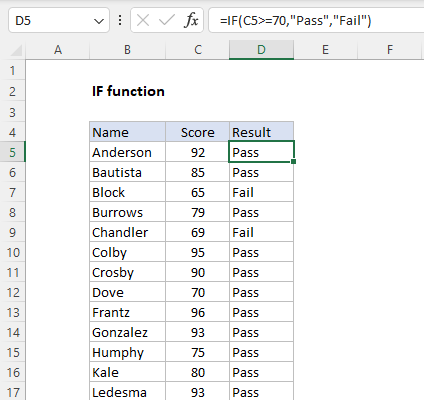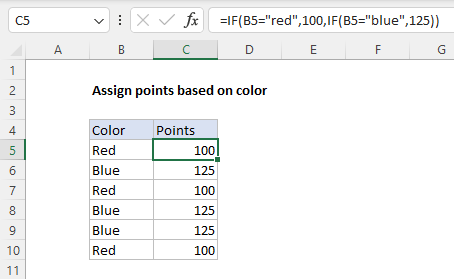Purpose
Test for a specific condition

Return value
The values you supply for TRUE or FALSE

Arguments
logical_test - A value or logical expression that can be evaluated as TRUE or FALSE.
value_if_true - [optional] The value to return when logical_test evaluates to TRUE.
value_if_false - [optional] The value to return when logical_test evaluates to FALSE.

Syntax
=IF(logical_test, [value_if_true], [value_if_false])

Notice that text values like "OK", "Yes", "No", etc. must be enclosed in double quotes (""). However, numeric values should not be enclosed in quotes.
=IF(A1>75,TRUE) // returns TRUE
=IF(A1>75,"OK") // returns "OK"
=IF(A1>85,"OK") // returns FALSE
=IF(A1>75,10,0) // returns 10
=IF(A1>85,10,0) // returns 0
=IF(A1>75,"Yes","No") // returns "Yes"
=IF(A1>85,"Yes","No") // returns "No"

In the worksheet shown above, we want to assign either "Pass" or "Fail" based on a test score. A passing score is 70 or higher. The formula in D6, copied down, is:
=IF(C5>=70,"Pass","Fail")

Note that the logical flow of this formula can be reversed. This formula returns the same result:
=IF(C5<70,"Fail","Pass")

Assign points based on color
In the worksheet below, we want to assign points based on the color in column B. If the color is "red", the result should be 100. If the color is "blue", the result should be 125. This requires that we use a formula based on two IF functions, one nested inside the other. The formula in C5, copied down, is:
=IF(B5="red",100,IF(B5="blue",125))